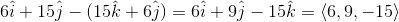# Calculus 3 : Normal Vectors

## Example Questions

### Example Question #71 : Normal Vectors

Find the normal vector to plane given by the equation of two vectors on the plane: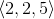and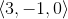.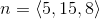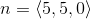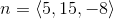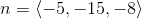Explanation:

To find the normal vector, you must take the cross product of the two vectors. Once you take the cross product, you get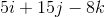. In vector notation, this is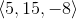.

### Example Question #72 : Normal Vectors

Calculate the norm of the vector: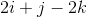None of the Above.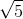Explanation:

Norm of the Vector is =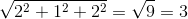### Example Question #73 : Normal Vectors

Two vectors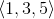and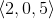are parallel to a plane. Find the normal vector to the plane.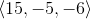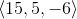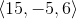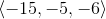Explanation:

To find the normal vector to the plane, we must take the cross product of the two vectors. Using the 3x3 matrix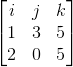, we perform the cross product.

Using the formula for the determinant of a 3x3 matrix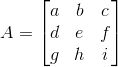is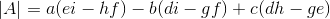,

we get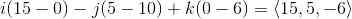### Example Question #74 : Normal Vectors

Find the normal vector of the plane that contains the lines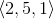and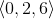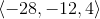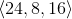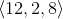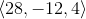Explanation:

To find the normal vector to the plane, you must the the cross (determinant) between the vectors .The formula for the determinant of a 3x3 matrixis. Using the matrix in the problem statement, we get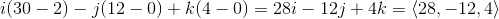### Example Question #75 : Normal Vectors

Find the normal vector to the plane given by the following vectors: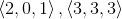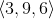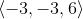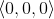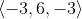Explanation:

The normal vector is given by the cross product of the vectors.

First, we must write the determinant in order to take the cross product of the two vectors: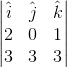where i, j, and k are the unit vectors corresponding to the x, y, and z direction respectively.

Next, we take the cross product. One can do this by multiplying across from the top left to the lower right, and continuing downward, and then subtracting the terms multiplied from top right to the bottom left: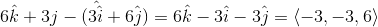### Example Question #76 : Normal Vectors

Find the normal vector to the plane that contains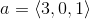and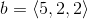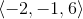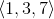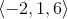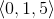Explanation:

The normal vector to the plane is found by taking the cross product ofand. Using the formula for taking the cross product of two vectors, where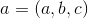and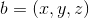, we get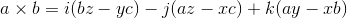. Using the vectors from the problem statement, we then get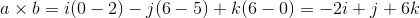. In vector notation, this becomes.

### Example Question #77 : Normal Vectors

Find the normal vector to the plane containing the following vectors: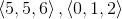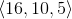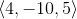Explanation:

The normal vector to the plane is given by the cross product of the two vectors in the plane.

First, we can write the determinant in order to take the cross product of the two vectors: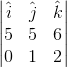where i, j, and k are the unit vectors corresponding to the x, y, and z direction respectively.

Next, we take the cross product. One can do this by multiplying across from the top left to the lower right, and continuing downward, and then subtracting the terms multiplied from top right to the bottom left: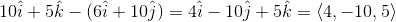### Example Question #78 : Normal Vectors

Find the normal vector to the plane containing the following vectors: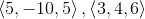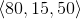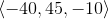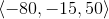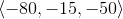Explanation:

The normal vector to the plane is given by the cross product of the two vectors in the plane.

First, we can write the determinant in order to take the cross product of the two vectors: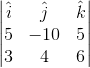where i, j, and k are the unit vectors corresponding to the x, y, and z direction respectively.

Next, we take the cross product. One can do this by multiplying across from the top left to the lower right, and continuing downward, and then subtracting the terms multiplied from top right to the bottom left: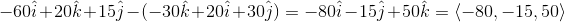### Example Question #79 : Normal Vectors

Find the normal vector to the plane given by the following vectors: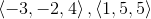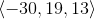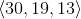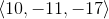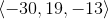Explanation:

The normal vector to the plane is given by the cross product of two vectors in the plane.

First, we must write the determinant in order to take the cross product of the two vectors: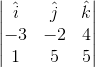where i, j, and k are the unit vectors corresponding to the x, y, and z direction respectively.

Next, we take the cross product. One can do this by multiplying across from the top left to the lower right, and continuing downward, and then subtracting the terms multiplied from top right to the bottom left: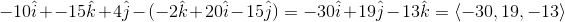### Example Question #80 : Normal Vectors

Find the normal vector to the plane given the vectors in the plane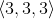and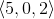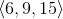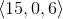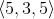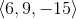Explanation:

To determine the normal vector to the plane, we must take the cross product of the two vectors in the plane.

First, we must write the determinant in order to take the cross product of the two vectors: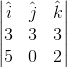where i, j, and k are the unit vectors corresponding to the x, y, and z direction respectively.

Next, we take the cross product. One can do this by multiplying across from the top left to the lower right, and continuing downward, and then subtracting the terms multiplied from top right to the bottom left: